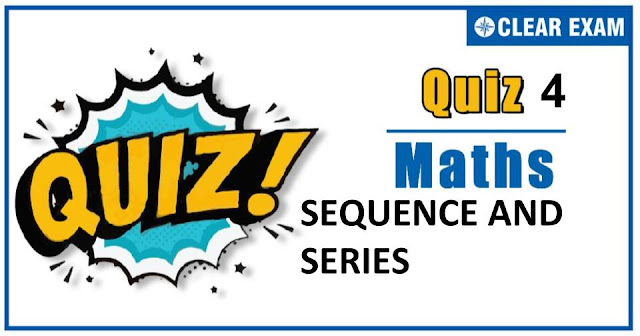## [LATEST]\$type=sticky\$show=home\$rm=0\$va=0\$count=4\$va=0

As per analysis for previous years, it has been observed that students preparing for JEE MAINS find Mathematics out of all the sections to be complex to handle and the majority of them are not able to comprehend the reason behind it. This problem arises especially because these aspirants appearing for the examination are more inclined to have a keen interest in Mathematics due to their ENGINEERING background.

Furthermore, sections such as Mathematics are dominantly based on theories, laws, numerical in comparison to a section of Engineering which is more of fact-based, Physics, and includes substantial explanations. By using the table given below, you easily and directly access to the topics and respective links of MCQs. Moreover, to make learning smooth and efficient, all the questions come with their supportive solutions to make utilization of time even more productive. Students will be covered for all their studies as the topics are available from basics to even the most advanced.

Q1. If a,b,c be in A.P., b,c,d are in G.P., and c,d,e are in H.P., then a,c,e will be in
•  A.P
•  G.P
•  H.P
•  None of these
Solution
We have, 2 b=a+c,c^2=bd and d=(2 ce)/(c+e) ∴c^2=((a+c)/2)d and d=(2 ce)/(c+e) ⇒c^2=((a+c)/2)((2 ce)/(c+e)) ⇒c^2=ae ⇒a,c,e are in G.P.

Q2.The arithmetic mean of 7 consecutive integers starting with a is m. Then, the arithmetic mean of 11 consecutive integers starting with a+2 is
•  2a
•  2m
•  a+4
•  m+4
Solution
(a+(a+1)+(a+2)+...+(a+6))/7=m ⇒7a+21=7m ⇒a+3=m ∴ ((a+2)+(a+3)+(a+4)+⋯+(a+12))/11 =(11a+77)/11 =a+7 =m-3+7 =m+4

Q3.  If the 7^th term of an H.P. is 1/10 and 12th term is 1/25, then 20th term is
•   1/37
•  1/41
•  1/45
•  1/49
Solution

Q4. If 1/a+1/c+1/(a-b)+1/(c-b)=0 and b≠a+c, then a,b,c are in
•  G.P
•  H.P
•  A.P
•  None of these
Solution
(b) We have, 1/a+1/c+1/(a-b)+1/(c-b)=0 ⇒1/a+1/(c-b)=1/(b-a)-1/c ⇒(a+c-b)/(a(c-b))=(c-b+a)/(c(b-a)) ⇒a(c-b)=c(b-a) ⇒ac-ab=bc-ac ⇒2ac=ab+bc⇒2ac/(a+c)=b⇒a,b,c are in H.P.

Q5.The sum of the integers from 1 to 100 which are not divisible by 3 or 5 is
•  2489
•  4735
•  2317
•  2632
Solution
Let S=1+2+3+...+100 =100/2 (1+100)=50(101)=5050 Let S_1=3+6+9+12+...+99 =3(1+2+3+4+...+33) =3.33/2 (1+33)=99×17=1683 Let S_2=5+10+15+...+100 =5(1+2+3+...+20) =5.20/2 (1+20)=50×21=1050 Let S_3=15+30+45+...+90 =15(1+2+3+...+6) =15.6/2 (1+6)=45×7=315 ∴ Required sum =S-S_1-S_2+S_3 =5050-1683-1050+315=2632

Q6. 2/(3 !)+4/(5 !)+6/(7 !)+⋯ is equal to
•  e^(1/2)
•  e^(-1)
•  e
•  e^(-1/3)
Solution
We have, 2/3!+4/5!+6/7!+⋯to ∞ =∑_(n=1)^∞▒2n/((2n+1))=∑_(n=1)^∞▒((2n+1))/(2n+1)!=∑_(n=1)^∞▒{1/2n!-1/(2n+1)!} =1/2!-1/3!+1/4!-1/5!+1/6!-1/7!+⋯to ∞ =e^(-1)

Q7.The sum to n terms of the infinite series 〖1.3〗^2+〖2.5〗^2+〖3.7〗^2+...∞ is
•  n/6 (n+1)(6n^2+14n+7)
•  n/6 (n+1)(2n+1)(3n+1)
•  4n^3+4n^2+n
•  None of the above
Solution
Given series is 〖1.3〗^2+〖2.5〗^2+〖3.7〗^2+...∞ This is an arithmetic-geometric series whose nth term is equal to T_n=n(2n+1)^2=4n^3+4n^2+n ∴S_n=∑_1^n▒T_n =∑_1^n▒〖(4n^3+4n^2+n)〗 =4∑_1^n▒n^3 +4∑_1^n▒n^2 +∑_1^n▒n =4(n/2 (n+1))^2+4/6 n(n+1)(2n+1)+n/2(n+1) =n(n+1)[n^2+n+4/6 (2n+1)+1/2] =n/6 (n+1)(6n^2+14n+7)

Q8.If a,b,c are in GP and x,y are arithmetic mean of a,b and b,c respectively, then 1/x+1/y is equal to
•  2/b
•  3/b
•  b/3
•  b/2
Solution
Given b^2=ac,x=(a+b)/2 and y=(b+c)/2 ∴ 1/x+1/y=2/(a+b)+2/(b+c) =(2(2b+a+c))/(ab+b^2+bc+ac) =(2(2b+a+c))/(ab+2b^2+bc) =(2(2b+a+c))/(b(2b+a+c)) =2/b

Q9.If log⁡a/(b-c)=log⁡b/(c-a)=log⁡c/(a-b), then a^a b^b c^c=
•  0
•  1
•  abc
•  None of these
Solution
We have, log⁡a/(b-c)=log⁡b/(c-a)=log⁡c/(a-b)=λ(say) ⇒a=10^(λ(b-c)),b=10^(λ(c-a)),c=10^(λ(a-b)) ∴a^a b^b c^c=10^(λ a(b-c) ).10^(λ b(c-a) ).10^(λ c(a-b)) ⇒a^a b^b c^c=10^(λ{a(b-c)+b(c-a)+c(a-b)})=10^(λ×0)=10^0=1

Q10. If x^log_x⁡〖(x^2-4x+5)〗 =(x-1), then x=
•  1
•  2
•  4
•  5
Solution
We have, x^log_x⁡〖(x^2-4x+5)〗 =x-1 ⇒x^2-4x+5=x-1⇒x^2-5x+6=0⇒x=2,3#### Written by: AUTHORNAME

AUTHORDESCRIPTION## Want to know more

Please fill in the details below:

## Latest NEET Articles\$type=three\$c=3\$author=hide\$comment=hide\$rm=hide\$date=hide\$snippet=hide

Name

ltr
item
BEST NEET COACHING CENTER | BEST IIT JEE COACHING INSTITUTE | BEST NEET, IIT JEE COACHING INSTITUTE: SEQUENCE AND SERIES QUIZ-4
SEQUENCE AND SERIES QUIZ-4
https://1.bp.blogspot.com/-Cx53XeZoyO0/X7EIha1A6pI/AAAAAAAAN0w/Q9N0LLbIn4oA7x8D8iUZf2WByUjaiaP8QCLcBGAsYHQ/w640-h336/Quiz%2BImage%2B20%2B%25283%2529.jpg
https://1.bp.blogspot.com/-Cx53XeZoyO0/X7EIha1A6pI/AAAAAAAAN0w/Q9N0LLbIn4oA7x8D8iUZf2WByUjaiaP8QCLcBGAsYHQ/s72-w640-c-h336/Quiz%2BImage%2B20%2B%25283%2529.jpg
BEST NEET COACHING CENTER | BEST IIT JEE COACHING INSTITUTE | BEST NEET, IIT JEE COACHING INSTITUTE
https://www.cleariitmedical.com/2020/11/sequence%20and%20series%20quiz%204.html
https://www.cleariitmedical.com/
https://www.cleariitmedical.com/
https://www.cleariitmedical.com/2020/11/sequence%20and%20series%20quiz%204.html
true
7783647550433378923
UTF-8